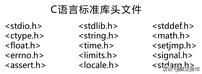# 常用math.h中的数学函数示例

C语言标准库C语言ctype.h中的字符判断函数

C语言string.h中的字符串函数

• sin(x)：x的正弦
• cos(x)：x的余弦
• tan (x): x的正切
• asin(x): x的反正弦
• acos( x): x的反余弦
• atan( x): x的反正切

• atan(x)：输入参数只有一个，取值范围为[-π/2, π/2]
• atan(y, x)：有两个输入参数，取值范围为[-π, π]

# 2、幂函数、指数函数和对数函数

• pow(x, y): x^y, x的y次方
• sqrt(x): x的平方根
• exp(x): 指数函数e ^x, e ≈ 2.718
• log(x): 自然对数函数 ln(x), x > 0
• log10(x): 以 10 为底的对数 log10(x), x > 0

# 3. 舍入函数

• ceil(x)：向上舍入，即不小于x的最小整数
• round( x)：四舍五入
• floor(x)：向下取整，即不大于x的最大整数

• fabs(x)：x的绝对值
• fmod( x , y): x/y的浮点余数
• ldexp(x, n): 计算x * 2^n的值

# 相关阅读

C语言标准库中包含的函数函数介绍（一）

C语言标准库中包含的函数介绍（三）